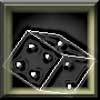#### You may also like### Gambling at Monte Carlo

A man went to Monte Carlo to try and make his fortune. Is his strategy a winning one?### Marbles and Bags

Two bags contain different numbers of red and blue marbles. A marble is removed from one of the bags. The marble is blue. What is the probability that it was removed from bag A?### Coin Tossing Games

You and I play a game involving successive throws of a fair coin. Suppose I pick HH and you pick TH. The coin is thrown repeatedly until we see either two heads in a row (I win) or a tail followed by a head (you win). What is the probability that you win?

# Distribution Differences

##### Age 14 to 16Challenge Level

Five Dice

We first consider rolling five regular dice, and finding the probability of the number of sixes which appear. Each roll is independent, so we are able to multiply the probabilities of "a six occuring" for each dice. For example,
\begin{equation*} Pr(\hbox{no sixes})=\left(\frac{5}{6}\right)^5 \end{equation*}
\begin{equation*} Pr(\hbox{all sixes})=\left(\frac{1}{6}\right)^5 \end{equation*}
When there are other results, say 3 sixes, we need to count the number of ways we can get a certain number of sixes from 5 dice. For example,
\begin{equation*} Pr(\hbox{3 sixes})=\pmatrix { 5 \cr 3}\left(\frac{5}{6}\right)^2\left(\frac{5}{6}\right)^2 \end{equation*}
This gives us the Binomial Distribution. However more importantly, we notice that we must get between 0 and 5 sixes. So the sum of these probabilities is 1. That is, our total event space is given by:
\begin{equation*} Pr(\hbox{1 six}) + ... + Pr(\hbox{5 sixes})=1, \end{equation*}

10cm Measurements

Now let us estimate 10cm, by writing two lines on a page which we think are 10 cm apart. Clearly, you and I would make different estimates of 10cm - but how different would these be?

Say we make measurements : {10.1, 10.0, 9.7, 9.9, 10.2, 11.8}

Just from a glance, we notice that 11.8cm is much larger than the other estimates. Indeed, we call this result an outlier. An important part in analysing statistics is understanding outliers and deciding when they can be ignored.

Now in our measurements of 10cm, do we expect to see more over-estimates, or under-estimates? There is no 'actual' difference between either, so we would expect our results to be symmetric and centred about the mean.

Random Variables

Let us stop briefly, to discuss what kind of random variables we are actually measuring. A random variable is a function that associates a unique numerical value with each outcome. For example, the number on the top of a tossed dice, or the length measured by a ruler.

Often we speak about continous and discrete random variables, and we will highlight their differences using the above two cases.

We know we are able to measure 10.01cm, 10.001cm, 10.0001cm, and so on, with our results mainly limited by the accuracy of our rulers.

On the other hand, it is impossible to have $4.2$ sixes, or $3\over 2$ fives. This is a physical constraint of our random variable, and shows that we can only have an integer number of sixes.

So in general, we see that a continuous variable can take a continuous range of values, however a discrete variable can only take a finite number of values (eg, integers). Note that in the case of estimating 10cm, we collect the data to the nearest mm. This makes our results discrete, even though the variable was itself continuous.

Values such as mean, variance, and mode, all depend on the type of random variable we are measuring. Although discrete and continuous variables seem quite distinct, it often necessary to model a continuous variable as a discrete one, or vice versa.

Indeed, it is worthwhile thinking what would happen if we added a discrete to a continuous variable...

Aces High

In Aces High, one hundred people each hold up the top card from their own pack. Independent of each person, we see that $Pr(\hbox{ace of spades})= {1\over 52}$. After the number of Ace of Spades has been counted, everyone puts their card at the bottom of their pack, and the turning process continues for a total of 52 times.

If we consider a person who gets the Ace of Spades on the 2nd turning, then this card will go to the back of the pile, and for the remaining 50 turnings they will not get the Ace of Spades. So the probability of an ace of spades appearing changes over time, which is unlike the Five Dice problem, where the $Pr(\hbox{six thrown})$ remains constant for all throws.

Conclusion

We conclude by noting the difference between population data, and sample data.

Measuring the height of all 11 year olds in England is virtually impossible, but estimating the mean height from a sample of 1000 is possible. However we need to remember that the data from any one random sample, will almost certainly be different to the data from another random sample. For example in Five Dice, you have a 1 in 7776 chance of repeating exactly the same rolls.

Analysing the distributions of the sample then allows us to estimate the true parameters of the population.This kind of mathematics leads to concepts such as the Normal/ Gaussian distribution and the Central Limit Theorem.